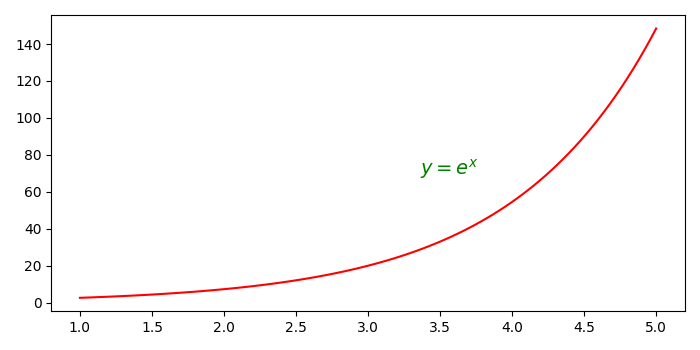# How to put text outside Python plots?

To put text outside a plot, we can change the text position by changing the value of text_pos_x and text_pos_y

## Steps

• Create data points for x and y.
• Initialize the text position of x and y.
• To plot x and y, use plot() method with color='red'.
• Use text() method to add text to figure.
• To display the figure, use show() method.

## Example

import numpy as np
from matplotlib import pyplot as plt
plt.rcParams["figure.figsize"] = [7.00, 3.50]
plt.rcParams["figure.autolayout"] = True
x = np.linspace(1, 5, 100)
y = np.exp(x)
text_pos_x = 0.60
text_pos_y = 0.50
plt.plot(x, y, c='red')
plt.text(text_pos_x, text_pos_y, "$\mathit{y}=e^{x}$", fontsize=14,
transform=plt.gcf().transFigure, color='green')
plt.show()

## Output